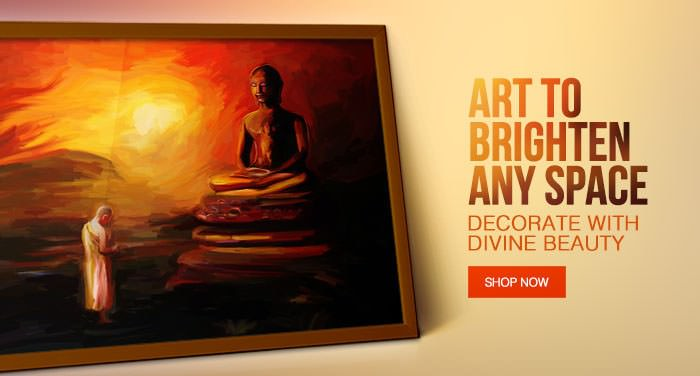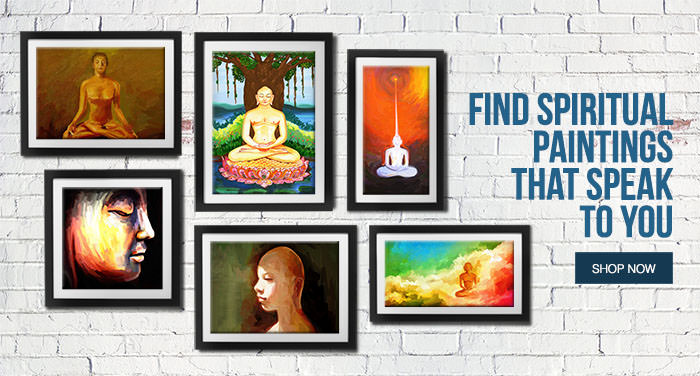# Jain ArtItems 21 to 40 of 52 total

Set Ascending Direction
per page

Grid  List

Page:

Starting at
Rs. 1,700.00

Starting at
Rs. 1,700.00

Starting at
Rs. 2,100.00

Starting at
Rs. 2,100.00

Starting at
Rs. 2,100.00

Starting at
Rs. 2,100.00

Starting at
Rs. 1,700.00

Starting at
Rs. 2,100.00

Starting at
Rs. 1,700.00

Starting at
Rs. 1,700.00

Starting at
Rs. 1,700.00

Starting at
Rs. 1,200.00

Starting at
Rs. 1,700.00

Starting at
Rs. 1,700.00

Starting at
Rs. 1,200.00

Starting at
Rs. 2,100.00

Starting at
Rs. 2,100.00

Starting at
Rs. 1,700.00

Starting at
Rs. 1,700.00
• ## The Wheel of Karma

Starting at
Rs. 1,700.00

Items 21 to 40 of 52 total

Set Ascending Direction
per page

Grid  List

Page: